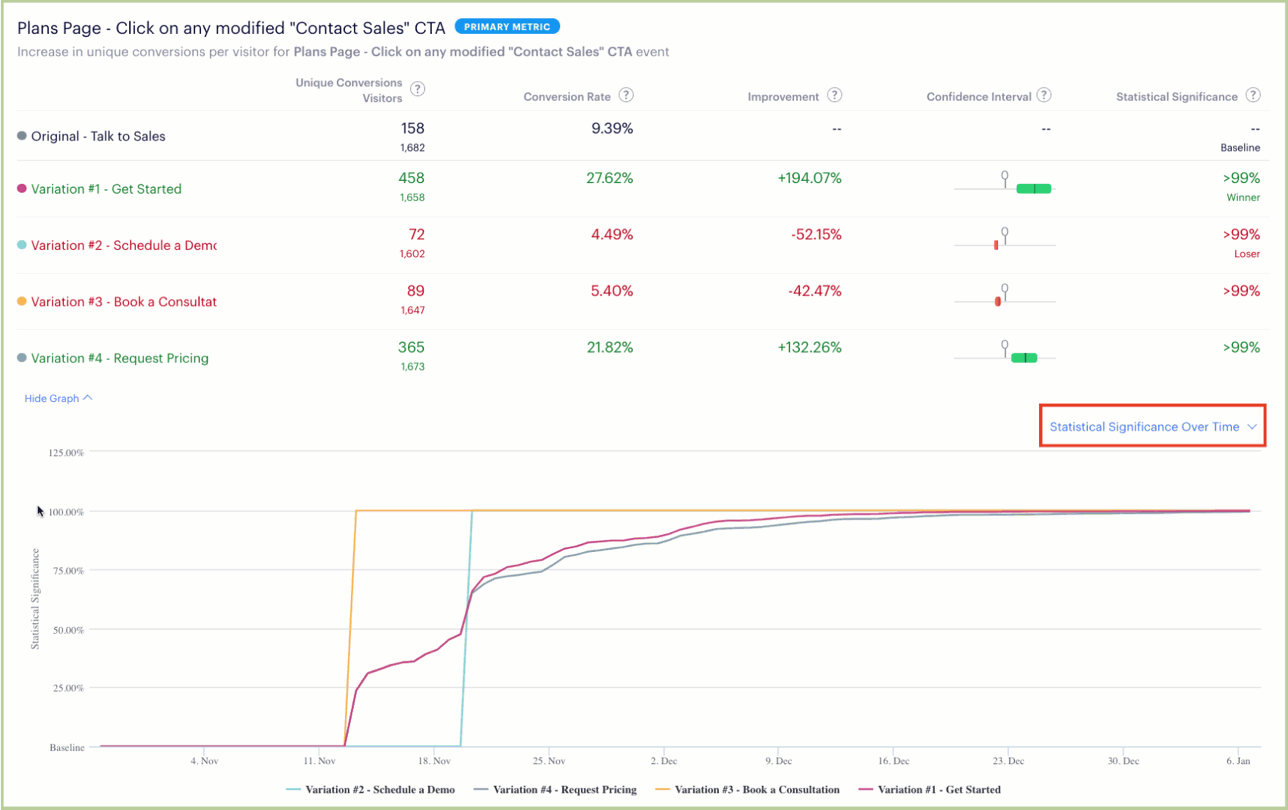# Learn Something New: Graph and Filter Experiment Results

We all know that data is great! But, there’s just so much available. Optimizely’s Results page provides graphs and filters such as date range, attributes (segmentation!), plus lets you select which variation acts as the Baseline to help you dig into results.

# Graphs

Optimizely helps you take your analysis to a new level by offering graphs to accompany the above statistics for each metric. These allow you to visualize trends and changes over time, even as the experiment is running, and then review them afterwards for deeper understanding. These graphs are interactive, so feel free to drag your cursor across the graph to view data at various points across the experiment run. Data points are updated to the results page within minutes and these graphs are updated when you update the time range or Segmentation of users. Additionally, results can be viewed in real time -- compared to a t-test where you would have to wait until your sample size has been reached to get meaningful results.

To view graphs, click the View Graph link below the data table under each metric. Then select which graph to display using the pull-down menu on the right.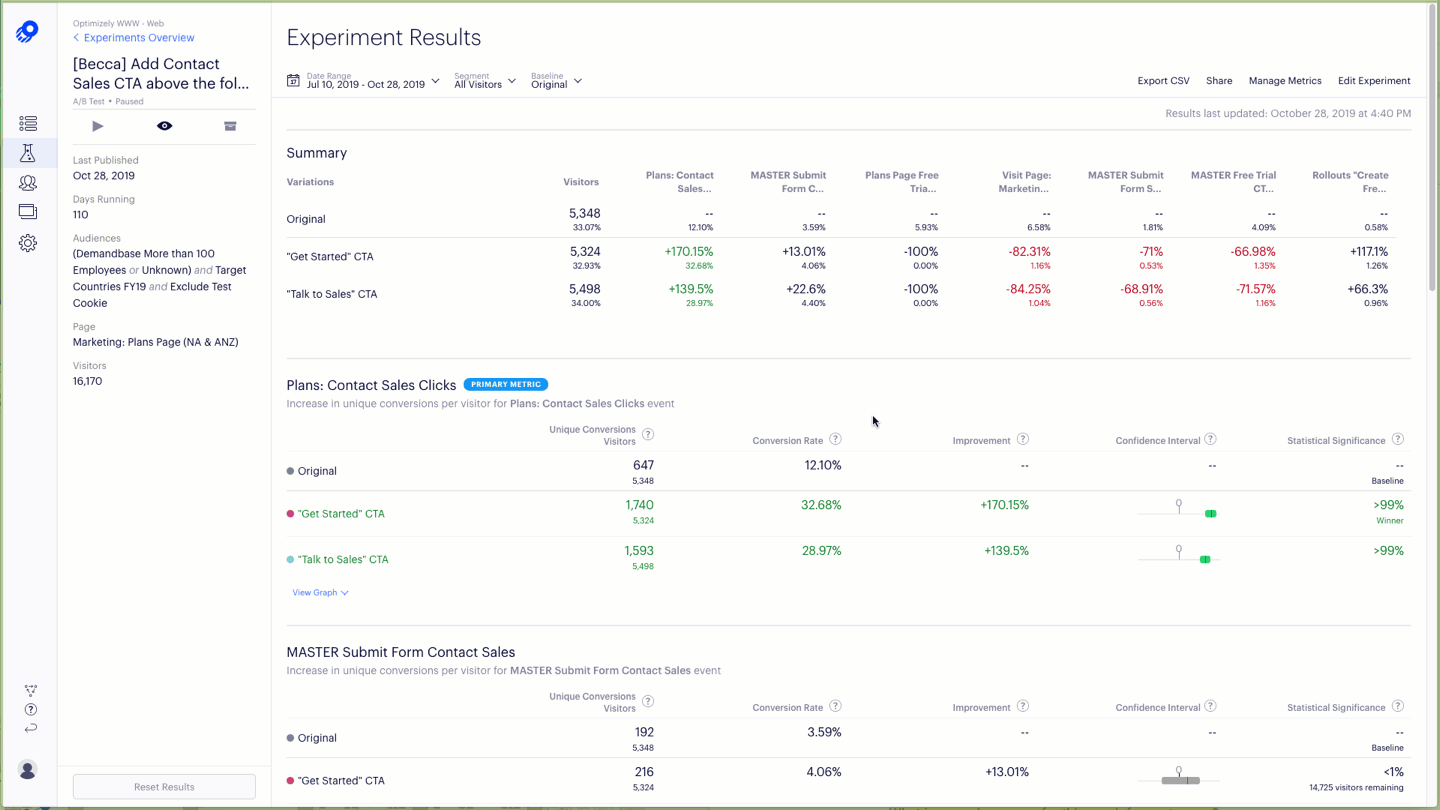## Graphs representing actual counts

The below two graphs have no calculations made.

Visitors Over Time - This is the simplest graph on the results page. Here each point represents the number of visitors bucketed into each variation and is displayed in an interactive time series. In other words, the line graph for each variation represents repeated measurements taken over regular time intervals. In the image below, you can easily see that weekday traffic is appreciably higher than weekend traffic on Opitmizely’s B2B site, not surprising for our vertical. In addition, you can use the graph to:

• Understand if your traffic allocation set up for variations is equal over time (especially if you made changes to it)
• Understand if you have had an influx of traffic during the experiment's lifecycle that may be influencing results
• Help determine a point-in-time where something may have broken in your experiment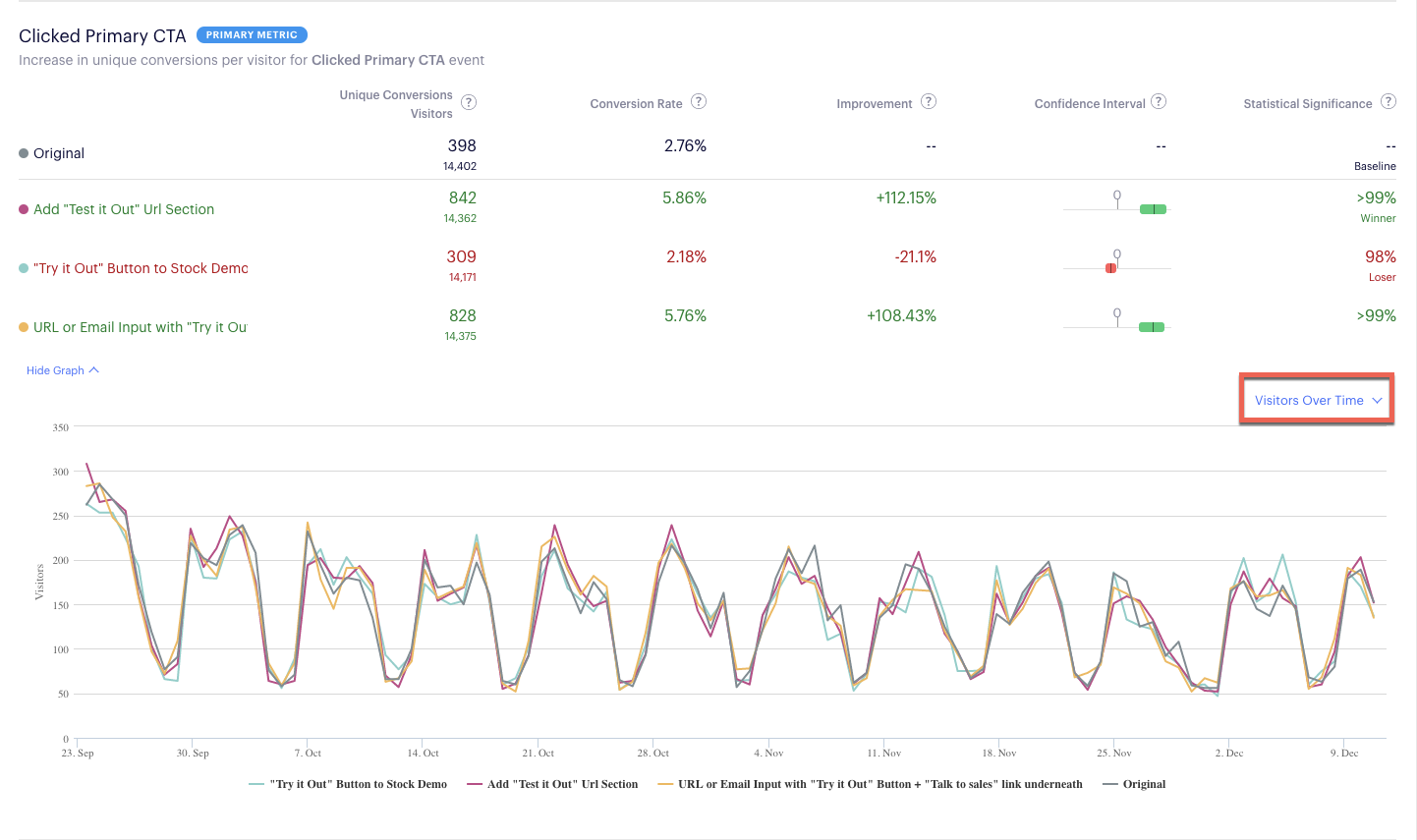Unique Conversions Over Time - Count of unique conversions per day in this metric for all variations and the original/baseline. This graph can help you:

• Understand if conversions have been consistent based on a week or day of the week
• Understand if an influx of traffic correlates to an increase in conversions at the same point-in-time. Spikes in Unique Conversions can help you identify if outliers may have skewed the data. If you suspect outliers are affecting results, take a look at our Outlier filtering in Optimizely article.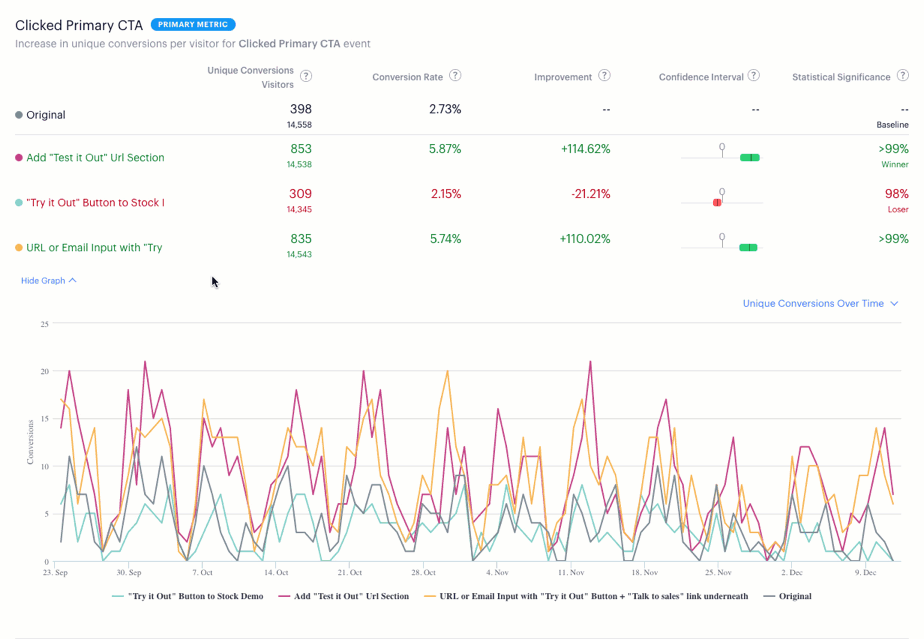## Graphs representing averages over a time series

These graphs take the raw data and present you with averages over time, where we compute the average of the first point, then the first two points, then the first three, and so on. This is known as the running average and is cumulative, which is why we can expect the graphs to stabilize to the true value (since the amount of data used in the calculation increases as we move right).

Conversion Rate Over Time - Using data from the first two graphs, Optimizely calculates to show the change in conversion rates for all variations and the original/baseline. The conversion rate is the percentage of unique visitors in the variation who triggered the metric/event. This graphs helps you:

• Determine if conversion rates have "normalized" both in trend and in disparity between the Original and the variation to help decide if a result is inconclusive (along with your sample size estimation)
• Make a determination on stopping a single variation if running multiple variations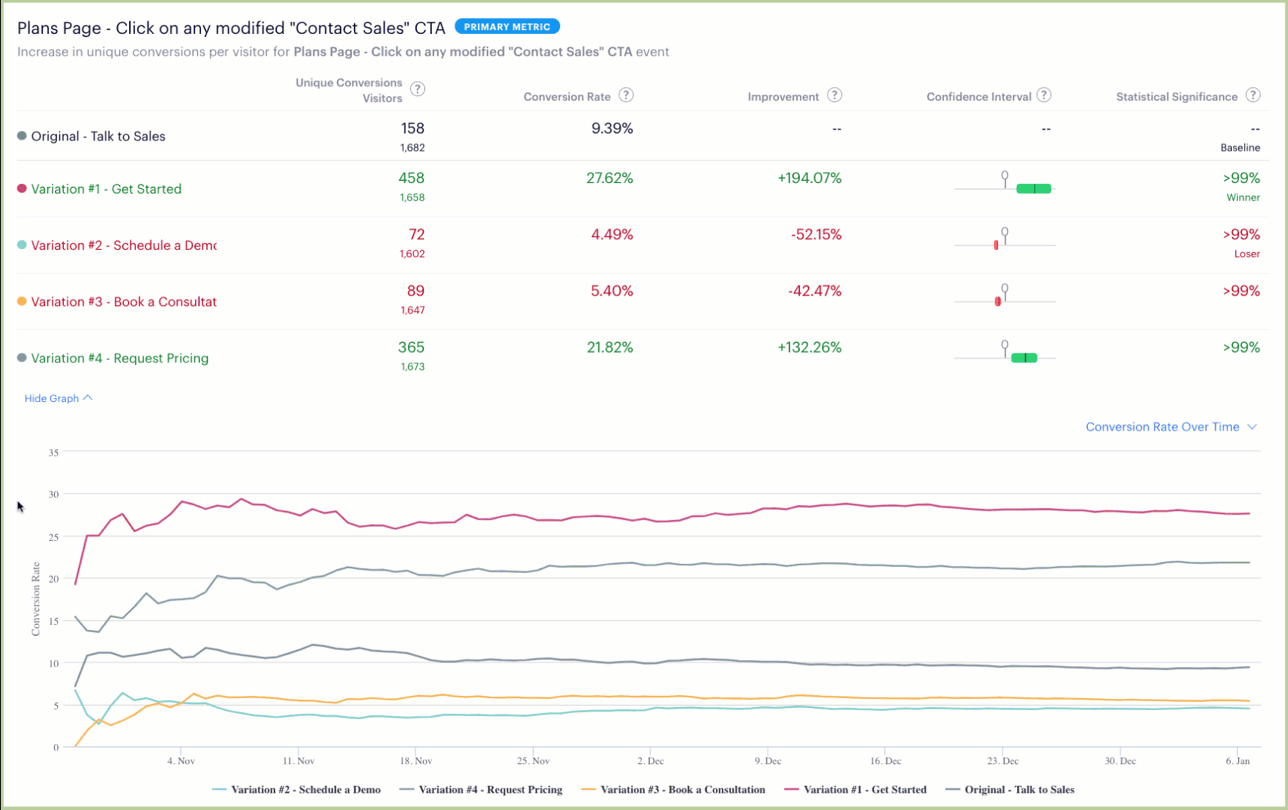Improvement Over Time - (the default) lays out the improvement for the variations compared to the baseline. To reduce confusion, the Results page in Optimizely shows the relative difference between variation and baseline measurement, not the absolute difference. This is true for all metrics, regardless of whether they are binary conversions or numeric.

In Optimizely, an improvement interval of 1% to 10% means that the variation sees between 1% and 10% improvement over baseline. For example, if the baseline conversion rate is 25%, you can expect the variation conversion rate to fall between 25.25% and 27.5%. The below graph helps you to:

• Understand how one of the primary data points for statistical significance is trending
• Understand your highest performing variations against the Original when running multiple variations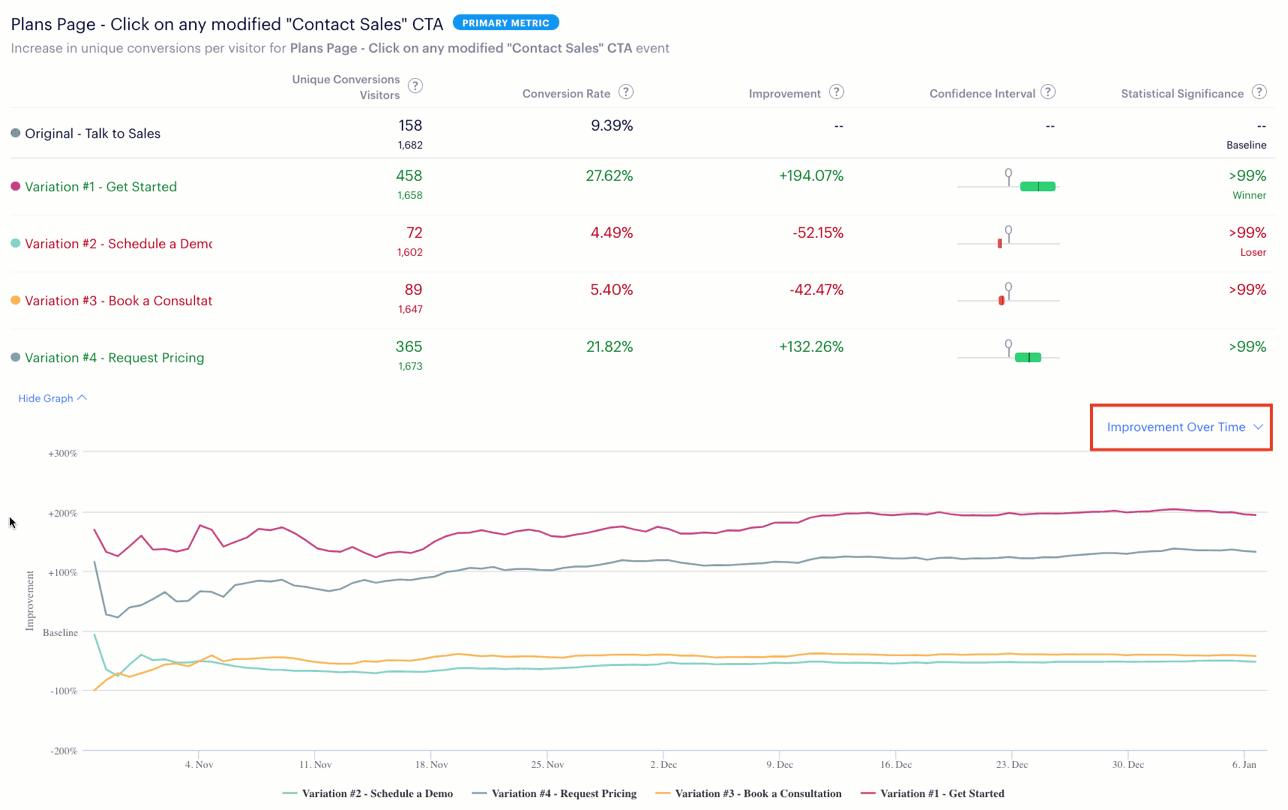Statistical Significance Over Time - Cumulative statistical significance for the variations. Note that significance and confidence intervals are still connected in the same way: your experiment reaches significance at exactly the same time that your confidence interval as improvement moves away from zero. This graphs help you:

• Understand if statistical significance has fluctuated to identify a point-in-time to investigate why
• Understand if there has been a "stats engine reset"